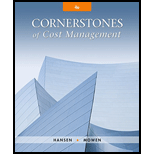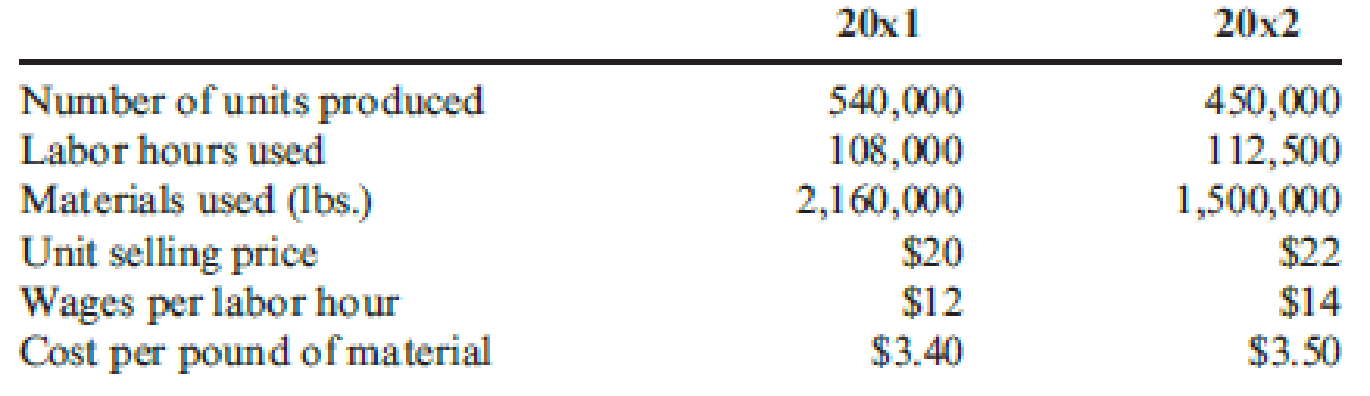# Refer to Cornerstone Exercise 15.3. Choctaw Company provides the following additional information so that total productivity can be valued: Required: 1. Calculate the cost of inputs in 20x2, assuming no productivity change from 20x1 to 20x2. 2. Calculate the actual cost of inputs for 20x2. What is the net value of the productivity changes? How much profit change is attributable to each input’s productivity change? 3. What if a manager wants to know how much of the total profit change from 20x1 to 20x2 is attributable to price recovery? Calculate the price-recovery component, and comment on its meaning.### Cornerstones of Cost Management (C...

4th Edition
Don R. Hansen + 1 other
Publisher: Cengage Learning
ISBN: 9781305970663

#### Solutions

Chapter
Section### Cornerstones of Cost Management (C...

4th Edition
Don R. Hansen + 1 other
Publisher: Cengage Learning
ISBN: 9781305970663
Chapter 15, Problem 4CE
Textbook Problem
27 views

## Refer to Cornerstone Exercise 15.3. Choctaw Company provides the following additional information so that total productivity can be valued:Required: 1. Calculate the cost of inputs in 20x2, assuming no productivity change from 20x1 to 20x2. 2. Calculate the actual cost of inputs for 20x2. What is the net value of the productivity changes? How much profit change is attributable to each input’s productivity change? 3. What if a manager wants to know how much of the total profit change from 20x1 to 20x2 is attributable to price recovery? Calculate the price-recovery component, and comment on its meaning.

1.

To determine

Compute the cost of inputs for the profile 20x2, if there is no change from productivity profile 20x1 to 20x2.

### Explanation of Solution

Productivity measurement: The productivity measurement refers to the quantitative assessment of the changes in the productivity.

Profile measurement and analysis: The profile measurement and analysis refers to the computation of a set of operational partial productivity measures and their comparison to the corresponding set of base period, for the assessment of the nature of changes in productivity.

Calculate the cost of inputs for the profile 20x2:

 Particulars Amount ($) Cost of labor (3)$1,260,000 Cost of materials (4) $6,300,000 Total PQ Cost$7,560,000

Table (1)

Working Notes (1):

Compute the base period productivity ratios for profile 20x1:

Compute the Labor Productivity Profile for 20x1:

Labor Productivity Profile = Number of Units producedLabor hours used=$540,000108,000 hours5.00 Working Notes (2): Compute the Material Productivity Profile for 20x1: Material Productivity Profile = Number of Units producedMaterials used=$540,0002,160,000 lbs0

2.

To determine

Compute the actual cost of inputs for the profile 20x2. Indicate the net value of productivity changes and identify the profit change attributable to productivity change of each unit.

3.

To determine

Compute and describe the price recovery component.

### Still sussing out bartleby?

Check out a sample textbook solution.

See a sample solution

#### The Solution to Your Study Problems

Bartleby provides explanations to thousands of textbook problems written by our experts, many with advanced degrees!

Get Started

Find more solutions based on key concepts
Classify consumer products

MKTG 12:STUDENT ED.-TEXT

An increase in accounts receivable is deducted from net income to compute cash from operating activities.

College Accounting, Chapters 1-27 (New in Accounting from Heintz and Parry)

EVA For 2014, Everyday Electronics reported 22.5 million of sales and 18 million of operating costs (including ...

Fundamentals of Financial Management, Concise Edition (with Thomson ONE - Business School Edition, 1 term (6 months) Printed Access Card) (MindTap Course List)

What are the main objectives of performance appraisal?

Foundations of Business (MindTap Course List)